# Magnetic Effects of Current and Magnetism   Share

## What is Magnetic Effects of Current and Magnetism

Magnetism has been known since the time of the ancient Greeks, but it has always been a bit mysterious. You can see electricity in the flash of a lightning bolt, but when a compass needle points to magnetic north, you can’t see any force causing it to rotate. You must have studied about electrostatic forces and fields, which are caused by electric charges at rest and these electric fields can move other free charges, such as by producing a current in a circuit. In this chapter, we will see that when an electric charge moves, it generates other forces and fields. These additional forces and fields are called magnetism.

Magnets are commonly found in everyday objects, such as toys, hangers, elevators, doorbells, and computer devices. Experiments on these magnets have shown that all magnets have two poles: One is labeled as North (N) and the other is labeled as South (S). Magnetic poles repel if they are alike (both N or both S) and attract if they are opposite (one N and the other S), and both poles of a magnet attract unmagnetized pieces of iron. An important point to note here is that you cannot isolate an individual magnetic pole. Every piece of a magnet, no matter how small, which contains a north pole must also contain a south pole.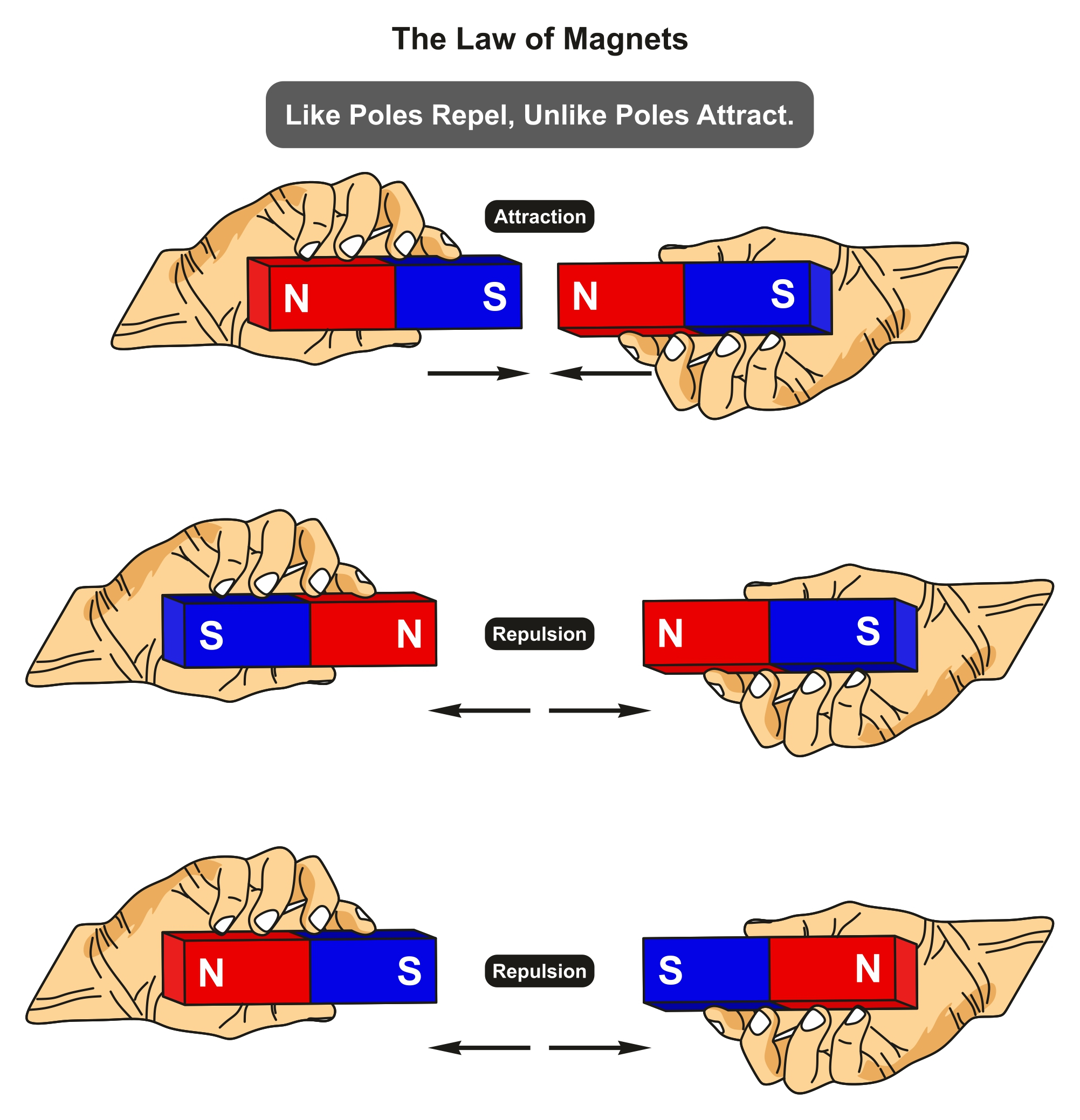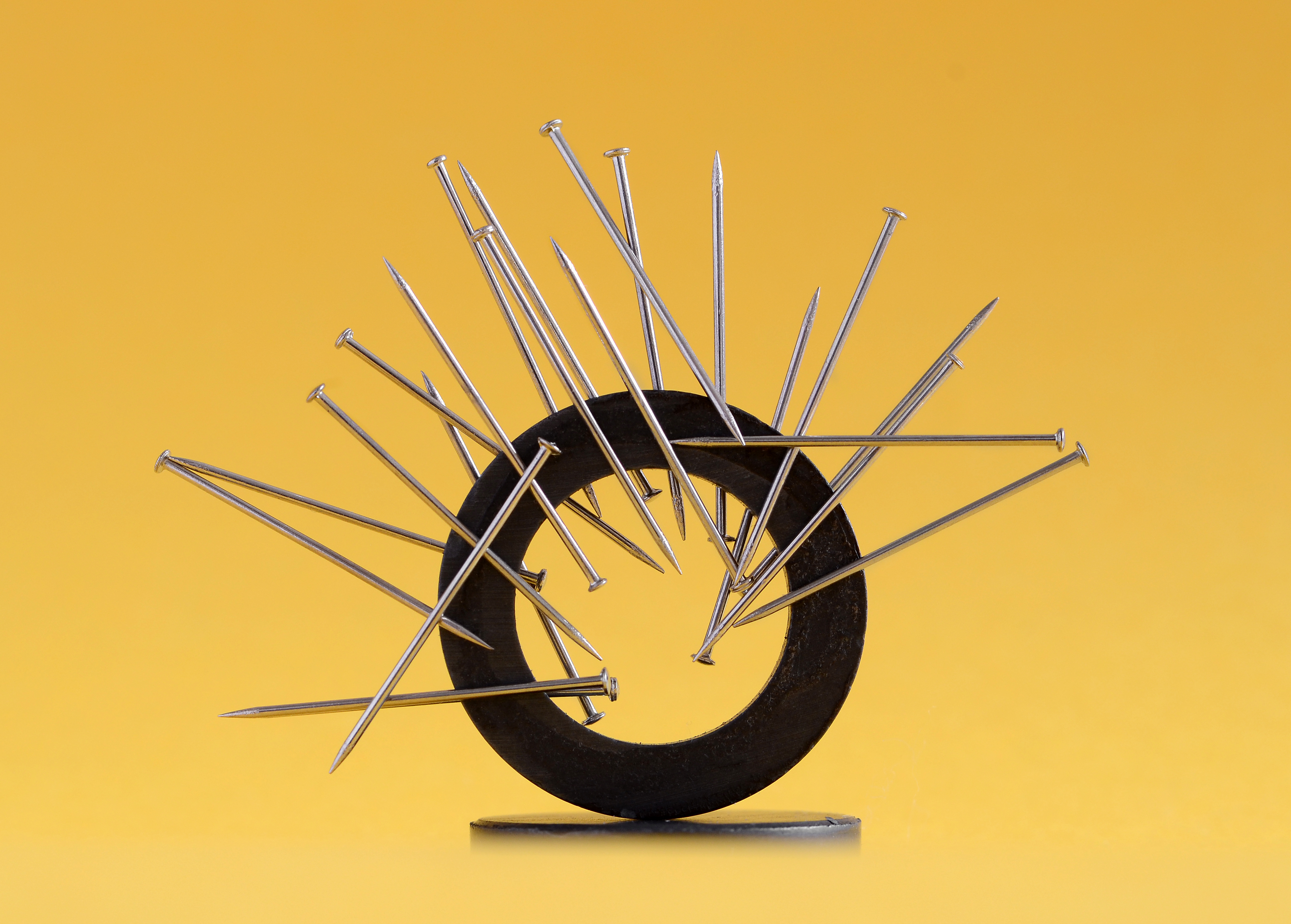In an experiment Danish physicist Hans Christian Oersted noticed that a current in a straight wire caused a deflection in a nearby magnetic compass needle. On experimenting further, it was observed that the alignment of the needle is tangential to an imaginary circle which has the straight wire as its centre and has its plane perpendicular to the wire. It was noticed that when the current is flowing in the wire, there is deflection in the needle and on reversing the direction of the current, the orientation of the needle also gets reversed. An increase in the deflection is observed on increasing the current or bringing the needle closer to the wire. If we sprinkle Iron filings around the wire, they arrange themselves in concentric circles with the wire. Oersted came to the conclusion that moving charges or currents produced a magnetic field in the surrounding space.

Prepare Online for JEE Main/NEET

Crack JEE 2021 with JEE/NEET Online Preparation Program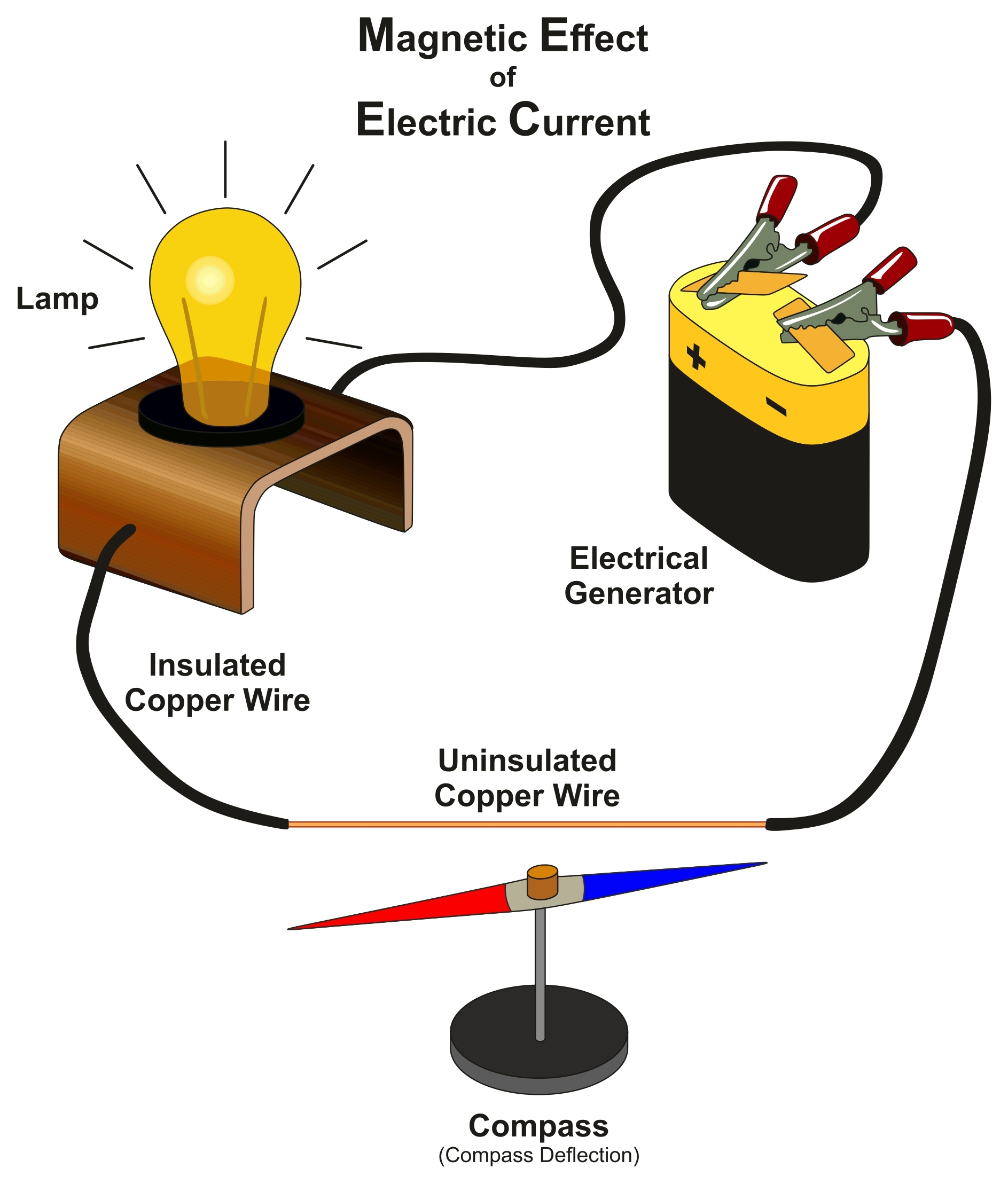## Notes for Magnetic Effects of Current and Magnetism

Now we will discuss each of the important topics along with an overview of the chapter followed by important formulas of the chapter which will help you in solving numerically related to Magnetic Effects of Current and Magnetism.

## Topics of Magnetic Effects of Current and Magnetism

• Biot - Savart law and its application

• Ampere's law and its applications

• Magnetic Force on a moving charge in uniform magnetic fields and electric fields

• Cyclotron

• Force on a current-carrying conductor in a uniform magnetic field

• The force between two parallel current-carrying conductors

• Torque experienced by a current carrying loop in a uniform magnetic field

• Moving coil galvanometer

• Current loop acting as a magnetic dipole and its magnetic dipole moment

• Para-, dia- and ferromagnetic substances

• Magnetic susceptibility and permeability

• Electromagnets and permanent magnets

## Overview of Magnetic Effects of Current and Magnetism

In this chapter, you will study how magnetic field exerts forces on moving charged particles, like electrons, protons, and current-carrying wires. You will also learn how currents produce magnetic fields and how particles can be accelerated to very high energies in a cyclotron. You will study how currents and voltages are detected by a galvanometer. You will also get to know about a convention that a current or a field (electric or magnetic) emerging out of the plane of the paper is depicted by a dot (?) and a current or a field going into the plane of the paper is depicted by a cross (⊗ ). You will also study that the direction of current is obtained using Right-hand thumb rule.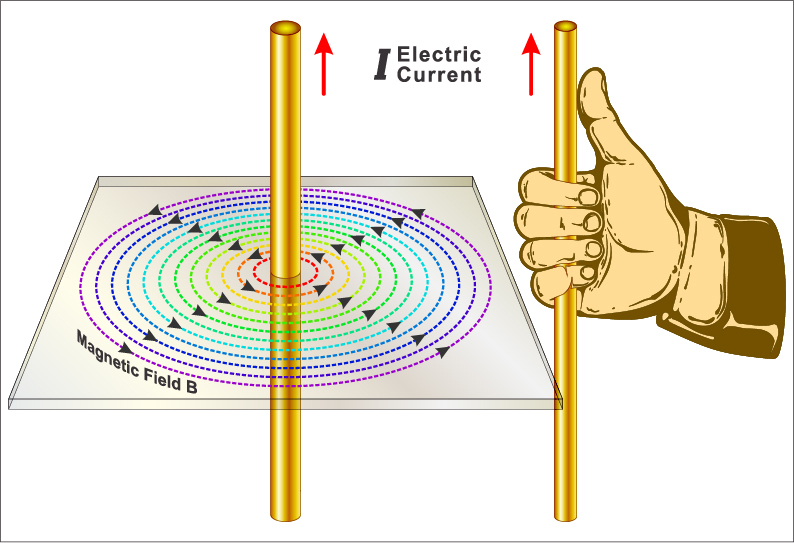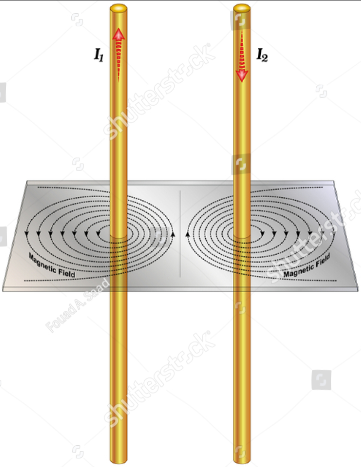You will study the representation of magnetic fields by magnetic field lines which is very useful in visualizing the strength and direction of the magnetic field. And each of these lines forms a closed loop. The field lines emerge from the north pole (N), loop around to the south pole (S), and continue through the bar magnet back to the north pole.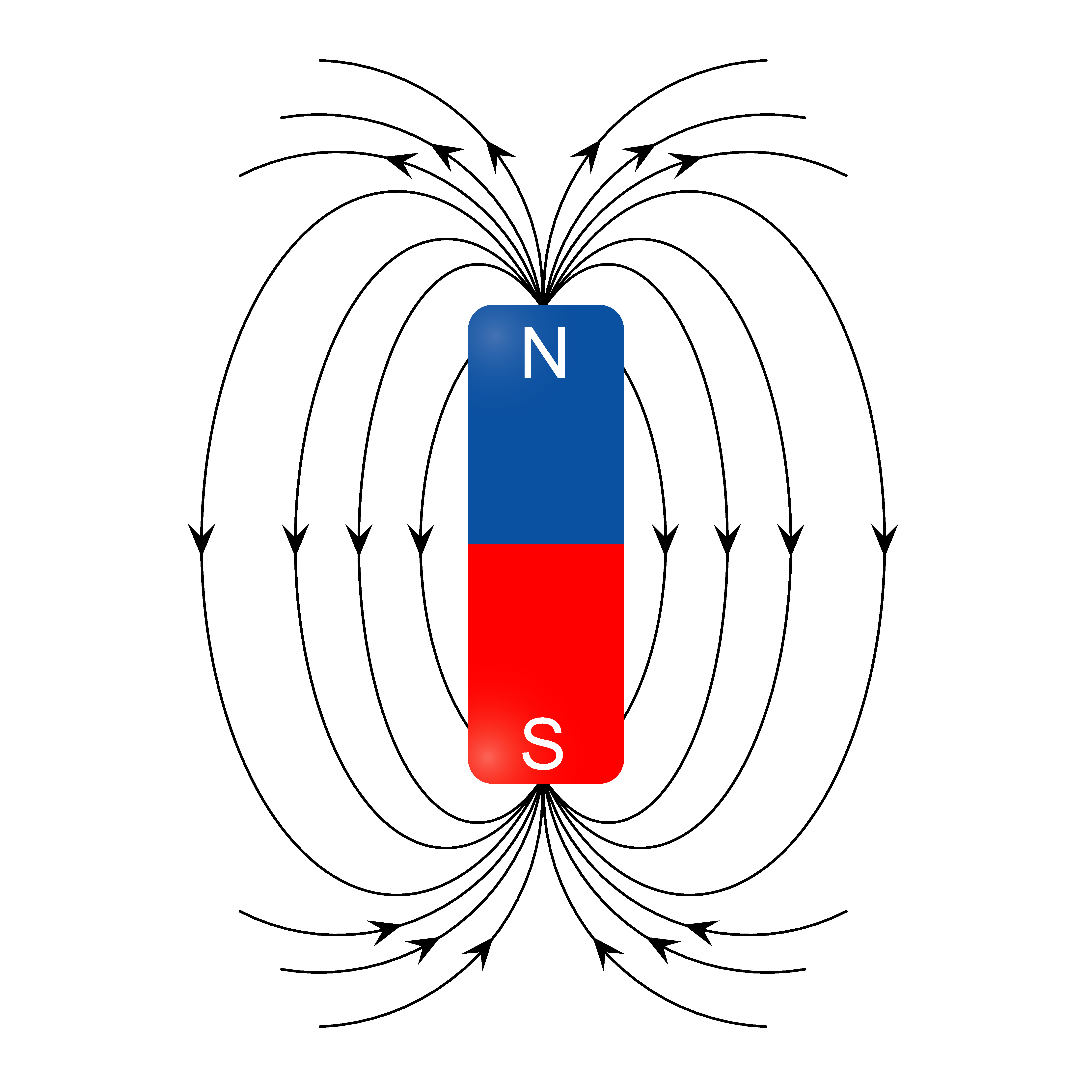We will discuss how magnetic fields are created by arbitrary distributions of electric current, using the BiotSavart law. Then we will look at how current-carrying wires create magnetic fields and will deduce the forces that arise between two current-carrying wires due to these magnetic fields. We also study the torques produced by the magnetic fields of current loops followed by generalizing these results to an important law of electromagnetism, called Ampère’s law. We will examine some devices that produce magnetic fields from currents in geometries based on loops, known as solenoids and toroids. Finally, we will look at how materials behave in magnetic fields and categorize materials based on their responses to magnetic fields.

## Formulas for Magnetic Effects of Current and Magnetism

1. Magnetic field due to Current Element:

2.  Magnetic field in vector form=:

3. Ampere's law=

4. Magnetic field Inside the solid cylinder=

5. Magnetic Field due to Circular Coil at the centre:

6. Magnetic field Inside the thick portion of hollow cylinder:

7. Magnetic Field at the axis due to circular current carrying wire:

8. Magnetic field in Infinite sheet carrying current:  , where  current density

9. Magnetic field in finite length solenoid:

10. Magnetic field in Toroid:

11. Force on a charged particle in a magnetic field:

12. The radius of charged particle:

13. Time period of charged particle:

14. Frequency of charged particle:

15. Lorentz Force:

## How to prepare for Magnetic Effects of Current and Magnetism

• First, you should have good command over the concepts of Magnetic Effects of Current and Magnetism and you should also know how to apply them well at the time of the entrance exam or while solving questions.
• Please try to understand each concept from this chapter, with the help of theory, questions with solutions and video lectures on each important concept. For each concept practice enough problems to have a thorough understanding of the concept.
• Solve all the questions at home with proper concentration and try to do all calculations by yourself without seeing the solution first. Understand the derivation of each formula very clearly because if you don't remember the formulas, you can easily derive them but try to remember all the formulas from this chapter because in certain questions you will get the answer by directly applying these formulas.
• Understand all the laws from this chapter along with their applications. With proper study, you can solve questions from this chapter with ease.

## Tips for Magnetic Effects of Current and Magnetism

1. Make a plan to prepare for this chapter and Stick to a Timetable.

2. Study the concept first and then start solving the question. Don't go through question directly without knowing the concept.

3. Solve previous year question from this chapter.

4. Practice as many questions as possible.

Because as said by Aristotle,

We are what we repeatedly do. Excellence, then, is not an act, but a habit.

## Books for Magnetic Effects of Current and Magnetism

For Magnetic Effects of Current and Magnetism, chapter concepts in NCERT are enough but you will have to practice lots of questions including previous year questions and you can follow other standard books available for competitive exam preparation like Concepts of Physics (H. C. Verma) and Understanding Physics by D. C. Pandey (Arihant Publications).

## Physics Chapter wise Notes For Engineering and Medical Exams

 Chapters No. Chapters Name Chapter 1 Physics and Measurement Chapter 2 Kinematics Chapter 3 Laws of motion Chapter 4 Work Energy and Power Chapter 5 Rotational Motion Chapter 6 Gravitation Chapter 7 Properties of Solids and Liquids Chapter 8 Kinetic theory of Gases Chapter 9 Thermodynamics Chapter 10 Oscillations and Waves Chapter 11 Electrostatics Chapter 12 Current Electricity Chapter 14 Electromagnetic Induction and Alternating currents Chapter 15 Electromagnetic Waves Chapter 16 Optics Chapter 17 Dual Nature of Matter and Radiation Chapter 18 Atoms And Nuclei Chapter 19 Electronic devices Chapter 20 Communication Systems Chapter 21 Experimental skills

### Topics from Magnetic Effects of Current and Magnetism

• Biot Savart law and its application to current carrying circular loop. ( JEE Main, COMEDK UGET, KEAM ) (17 concepts)
• Bar magnet as an equivalent solenoid, magnetic field lines ( JEE Main, COMEDK UGET, KEAM ) (62 concepts)
• Ampere's law and its applications to infinitely long current carrying straight wire and solenoid. ( JEE Main, COMEDK UGET, KEAM ) (4 concepts)
• Current loop as a magnetic dipole and its magnetic dipole moment. ( JEE Main, COMEDK UGET, KEAM ) (27 concepts)
• Force on a moving charge in uniform magnetic and electric fields, Cyclotron ( JEE Main, COMEDK UGET, KEAM ) (27 concepts)
• Force on a current-carrying conductor in a uniform magnetic field. ( JEE Main, COMEDK UGET, KEAM ) (13 concepts)
• The force between two parallel current-carrying conductors-definition of the ampere. ( JEE Main, COMEDK UGET, KEAM ) (2 concepts)
• Earth's magnetic field and magnetic elements. Para-, dia- and ferro- magnetic substances. ( JEE Main, COMEDK UGET, KEAM ) (48 concepts)
• Torque experienced by a current loop in uniform magnetic field ( JEE Main, COMEDK UGET, KEAM ) (10 concepts)
• Moving coil galvanometer, its current sensitivity, and conversion to ammeter and voltmeter. ( JEE Main, COMEDK UGET, KEAM ) (11 concepts)
• Magnetic susceptibility and permeability, Hysteresis ( JEE Main, COMEDK UGET, KEAM ) (15 concepts)
• Electromagnets and permanent magnets. ( JEE Main, COMEDK UGET, KEAM ) (5 concepts)
• Biot-Savart Law and its applications ( JEE Main, COMEDK UGET, KEAM ) (4 concepts)
• Ampere's law and its applications ( JEE Main, COMEDK UGET, KEAM ) (5 concepts)
• Motion of a charged particle in uniform magnetic and electric fields ( JEE Main, COMEDK UGET, KEAM ) (5 concepts)
• Magnetism and matter ( JEE Main, COMEDK UGET, KEAM ) (8 concepts)
• Magnetic force on a current carrying conductor ( JEE Main, COMEDK UGET, KEAM ) (2 concepts)
• Torque on a current loop, Magnetic Dipole ( JEE Main, COMEDK UGET, KEAM ) (3 concepts)
• Moving coil galvanometer ( JEE Main, COMEDK UGET, KEAM ) (1 concepts)

### Important Books for Magnetic Effects of Current and Magnetism

•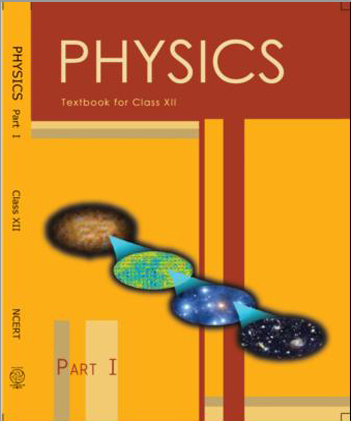•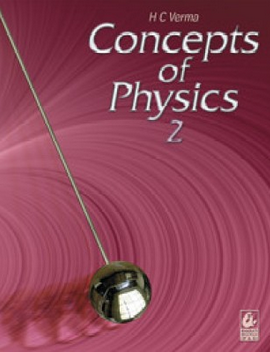•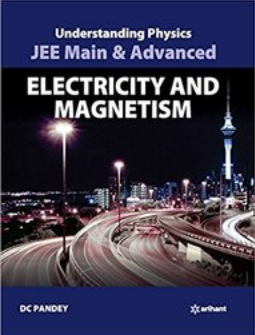Exams
Articles
Questions